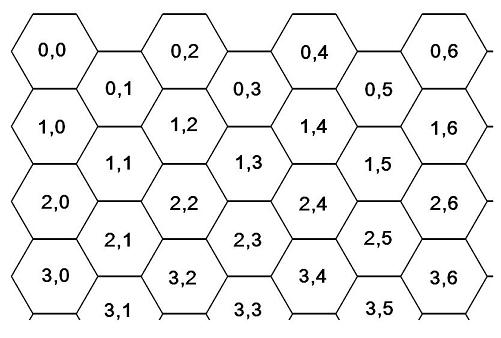Honey Bees
Tag(s):
No tags
Problem
Editorial
Analytics

The Queen Bee has asked the Worker Bees to write a program to answer queries regarding her T hives. A hive can be visualized using a rectangular matrix of size N X M . Figure below shows the coordinates of each cell. Each cell contains honey which is depicted by a number in the corresponding 2D array ( <= 100).The Queen Bee asks Q queries which need to be answered, they are of the following types :

1 X Y -- To sum the honey over all cells which are at a 1-hop distance from cell (X, Y) i.e. it takes only 1 hop in any direction from cell (X, Y) to reach these

2 X Y -- To sum the honey over all cells which are at a 2-hop distance from cell (X, Y) i.e. it takes only 2 hops in any direction from cell (X, Y) to reach these

A hop is defined as moving from a cell (X, Y) to a cell it shares a common edge with i.e. its immediate neighbor.

You'll be given T, the number of testcases, followed by N, M, the size of the matrix/hive. The next N lines contain M integers each consisting the honey amount in each cell. This is followed by Q, the number of queries. The next Q lines are of the type 1 X Y and 2 X Y where (X, Y) is the cell location.

Output every query in a new line.

1 <= T <= 10

1 <= N, M, Q <= 200

0 <= X < N and 0 <= Y < M

Problem Setter - Dhvit Mehta

SAMPLE INPUT
1
4 4
1 2 3 4
5 6 7 8
9 1 3 4
1 2 3 4
2
1 0 1
2 0 1
SAMPLE OUTPUT
22
25
Explanation

For the first query,

The cells at a 1-hop distance from (0, 1) are (0, 0), (1, 0), (1, 1), (1, 2) and (0, 2)

For the second query

The cells at a 2-hop distance from (0, 1) are (2, 0), (2, 1), (2, 2), (1, 3) and (0, 3)

Time Limit: 0.6 sec(s) for all input files combined.
Memory Limit: 256 MB
Source Limit: 1024 KB
Marking Scheme: Marks are awarded when all the testcases pass.
Allowed Languages: Bash, C, C++, C++14, C++17, Clojure, C#, D, Erlang, F#, Go, Groovy, Haskell, Java, Java 8, Java 14, JavaScript(Rhino), JavaScript(Node.js), Julia, Kotlin, Lisp, Lisp (SBCL), Lua, Objective-C, OCaml, Octave, Pascal, Perl, PHP, Python, Python 3, Python 3.8, R(RScript), Racket, Ruby, Rust, Scala, Swift-4.1, Swift, TypeScript, Visual Basic

## CODE EDITOR

Initializing Code Editor...

## This Problem was Asked inChallenge Name

Codemania 2.0

OTHER PROBLEMS OF THIS CHALLENGE
• Algorithms > Dynamic Programming
• Math > Number Theory
• Algorithms > Dynamic Programming
• Algorithms > Graphs
• Basic Programming > Input/Output
• Data Structures > Disjoint Data Structures
• Basic Programming > Implementation